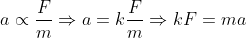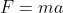Question 2: Apply Newton’s laws to explain the motion of objects in a variety of contexts.

## Newton’s First Law of Motion

“In the absence of an external unbalanced force, if a body is at rest, will remain at rest, and if in motion, will continue to do so forever.”  Mathematically, if Fnet = ∑F = 0 then a = 0.

### Newton's First Law of Motion and Law of Inertia

Inertia is the property of material objects which refers to their resistance of acceleration. This is the natural tendency of material objects to remain at rest or in motion with constant velocity. Quantitatively. Inertia is measured by the mass of the object.

Due to similarity of sense, Newton’s First Law is also called law of inertia. For example,

• By virtue of inertia a book on the table will remain there forever unless someone picks it. However, Newton’s law says the book is at rest because the force of gravity and normal reaction of the table surface balance one another. When a person picks it, he applies an additional unbalanced force which changes its state of rest.
• A ball kicked by the foot of a footballer comes to rest (and falls down) due to the unbalanced force of the resistance of the air (and force of gravity). If these unbalanced forces did not exist, the ball would continue to move with uniform velocity.

## Newton's First Law in different contexts

• A book on your study table or the table on the floor will remain on its respective places unless you pick them up or displace it.
• During earth quake, the tall building sometimes fall down. This is because the buildings are at rest and try to remain at rest. However, the quake sets the earth below them in motion. The bottom part of the buildings, more connected to the earth, moves with the earth and the buildings fall down as a consequence.

Above are two examples of the first part of the Newton’s First law.

• When a footballer hits the ball in the air, it stops and falls down due to friction with the air and gravity (Net or unbalanced force of Newton’s law). If these forces were not there the ball would go straight with the same velocity forever.
• When we ride a bicycle and stop pedaling, the bicycle goes for some time on its own. This is because the cycle wants to continue its motion. If the force of friction (unbalance force of Newton’s law) was not there, it would go straight with the same velocity forever.

The last two examples explain the second part of the Newton’s First Law.

## Newton's Second Law of Motion

An unbalanced force on a body produces acceleration in the body in its own direction which is directly proportional to the applied force and inversely proportional to the mass of the body. Mathematically, a ∝ F and a ∝ 1/m. Combining,Where k is the constant of proportionality and its value in SI system is 1. Therefore, mathematical form of Newton’s Second Law isExplanation: Both First and Second Law of Newton describe ‘force’. However, in First Law the description is qualitative (emphasis on the quality of force that it moves or stops a body) and the Second Law gives a quantitative measurement of the force, in newtons! Also we note that if the force produces acceleration in its own direction, then the velocity of an already moving body will further increase if the applied force and velocity of the body are in one direction. In such a case, the acceleration is positive. One the other hand, if the direction of the force is opposite to the direction of the velocity of the body, the applied force will slow down the velocity of the body. In such cases, the acceleration is said to be negative.

Unit of force: One interesting result of Newton’s Second Law is that it gives us the definition of the unit of force, newton. “The force is said to be 1 newton if it produces an acceleration of 1 ms-2 when applied to a body of 1 kg”.

## Newton's Second Law in different contexts

• A simple example is when a footballer kicks the ball, the ball moves in the direction in which it is kicked, i-e, in the direction of the applied force. It is also commonplace that if the if the ball is kicked with large force, it will move faster (more acceleration) and vice versa.
• Newton’ 2nd law may also be explained in the context of racecar industry. We all have observed that the manufacturers of race-cars always try to make cars with lighter masses. This is because, by Newton’s Second Law, the acceleration of the car is inversely proportional to the applied force of engine. So, reducing the mass considerably increases the acceleration of the racecar.

## Newton's Third Law of Motion

To every action, there is always an equal and opposite reaction.

If a body A exerts a force FAB on body B, then as a reaction, body B will exert a force FBA on body B. The forces will be equal in magnitude; however, the direction of the reaction force will be opposite to the direction of action force.This is the mathematical form of the law.

Newton’s 3rd law has some important applications.

• Action and reaction forces always occur in pair of equal magnitudes and opposite directions. So, interestingly no body can experience a force from the surrounding until this body does not exert force on the surrounding itself. Thus, a single isolated force does not exist.
• Action force acts on one body and reaction force on the another, therefore, they do not cancel each other.

## Newton's Third Law in different contexts

• While walking, how easily we take the step forward. This is because we push the earth backward with our foot and as a reaction the earth pushes us forward and we take our step forward (normally, our leg has enough weight).
• When we fire gun, we feel a recoil back. The gun acts on the bullet to go forward. As a reaction, the bullet pushes the gun backward.
• Fuel burns in the combustion chamber of the rocket and gases are ejected downward with a certain force. As a reaction, the gas exerts force on the rocket and pushes it up.